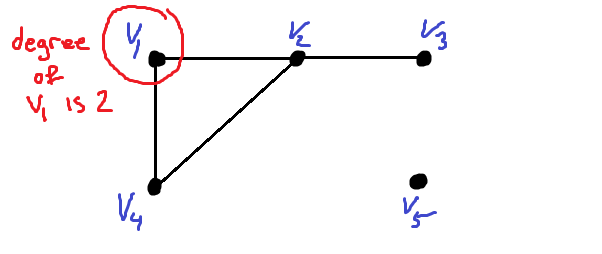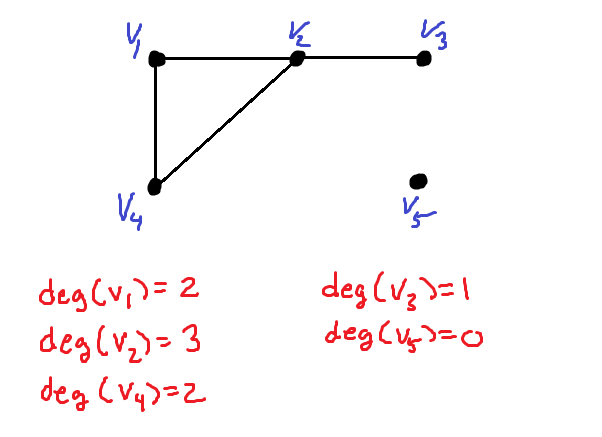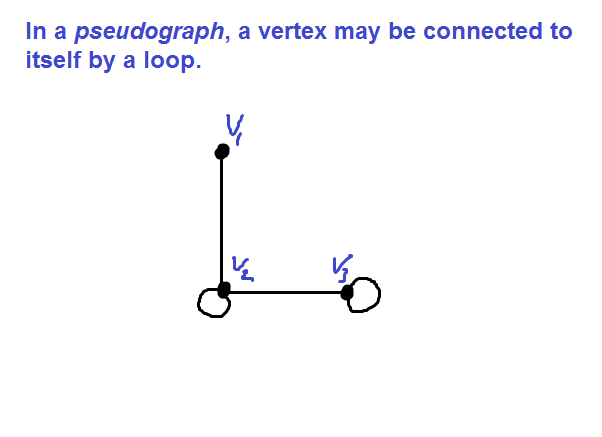﻿ The degree of a vertex in an undirected graph - MathBootCamps

# The degree of a vertex in an undirected graph

In graph theory, a graph consists of vertices and edges connecting these vertices (though technically it is possible to have no edges at all.) The degree of a vertex represents the number of edges incident to that vertex. In this lesson, we will explore what that means with examples and look at different cases where the degree might not be as simple as you would guess.

## The degree of a vertex in a simple graph

A simple graph is the type of graph you will most commonly work with in your study of graph theory. In these types of graphs, any edge connects two different vertices. An example of a simple graph is shown below.We can label each of these vertices, making it easier to talk about their degree. When you are trying to determine the degree of a vertex, count the number of edges connecting the vertex to other vertices.Consider first the vertex $$v_1$$. There are two edges incident with this vertex. Therefore, $$v_1$$ has degree 2.Using a common notation, we can write: $$\text{deg}(v_1) = 2$$.

In fact, the degree of $$v_4$$ is also 2. Vertex $$v_2$$ has 3 edges connected to it, so its degree is 3. Vertex $$v_3$$ has only one edge connected to it, so its degree is 1, and $$v_5$$ has no edges connected to it, so its degree is 0.Not all graphs are simple graphs. We still must consider two other cases: multigraphs and pseudographs.

## Multigraphs and the degree of a vertex

Multigraphs allow for multiple edges between vertices. An example of a multigraph is shown below.In a multigraph, the degree of a vertex is calculated in the same way as it was with a simple graph. In the graph above, the vertex $$v_1$$ has degree 3, since there are 3 edges connecting it to other vertices (even though all three are connecting it to $$v_2$$).We can now use the same method to find the degree of each of the remaining vertices.Hint: You can check your work by using the handshaking theorem. It states that the sum of all the degrees in an undirected graph will be 2 times the number of edges. In the example above, the sum of the degrees is 10 and there are 5 total edges.

## Pseudographs and the degree of a vertex

Pseudographs are not covered in every textbook, but do come up in some applications. These are graphs that allow a vertex to be connected to itself with a loop. In the example below, we see a pseudograph with three vertices. Vertex v2 and vertex v3 each have an edge connecting the vertex to itself.When calculating the degree of a vertex in a pseudograph, the loop counts twice.In the graph above, vertex $$v_2$$ has two edges incident to it. But, it also has a loop (an edge connecting it to itself). This adds 2 to the degree, giving this vertex a degree of 4. Similarly, $$v_3$$ has one edge incident with it, but also has a loop. Therefore its degree is 3.

Note that with this convention, the handshaking theorem still applies to the graph. There are 4 edges, since each loop counts as an edge and the total degree is:

$$1 + 4 + 3 = 8 = 2 \times \text{(number of edges)}$$

## Summary

The degree of a vertex is the number of edges incident to the vertex. This is simply a way of saying “the number of edges connected to the vertex”. It is common to write the degree of a vertex v as deg(v) or degree(v). If you are working with a pseudograph, remember that each loop contributes 2 to the degree of the vertex.